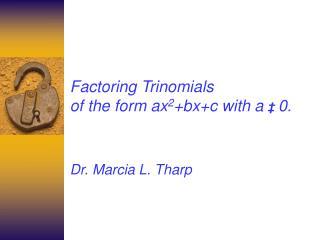DownloadDownload PresentationFactoring Trinomials of the form ax 2 +bx+c with a ‡ 0.

# Factoring Trinomials of the form ax 2 +bx+c with a ‡ 0.

Télécharger la présentation## Factoring Trinomials of the form ax 2 +bx+c with a ‡ 0.

- - - - - - - - - - - - - - - - - - - - - - - - - - - E N D - - - - - - - - - - - - - - - - - - - - - - - - - - -
##### Presentation Transcript

1. Factoring Trinomials of the form ax2+bx+c with a ‡0. Dr. Marcia L. Tharp

2. Why learn to factor trinomials? • Factoring trinomials is an important • Algebraic skill. It is used in higher-level mathematics and science. Besides that you will need it to simplify polynomial expressions and solve more complex equations.

3. Lesson 1 - Recognizing The Form ax2+bx+c with a ‡ 0 • The trinomials we will factor that have the form ax2+bx+c where a ‡ 0 and a, b, and c are real numbers. For example in ax2+bx+c Let a=1, b=2 and c=4 Then we get a trinomial 1x2+2x+4 Remember 1 x2 means x2. So we can write x2+2x+4 too which fits this form.

4. More ExamplesLet’s look at some more examples where we will replace the values a, b, and c in the form ax2+bx+c. If a=3, b=20 and c=12 do we get a trinomial that will fit our form that we will factor? This time we get a trinomial 3x2+20x+12 which fits the form we are going to try to factor. since a ‡ 0 and a, b, and c are real numbers.

5. What happens if we let a=4, b=0 and c=25? Will we get the trinomial form we need when we will replace the values a, b, and c in the form ax2+bx+c? Instead we get the binomial 4x2+25 which we will not factor here. It only has 2 terms so it is not a trinomial we are going to factor. This fits the form since a ‡0 where a, b, and c are real numbers. However, we won’t discuss this here since we have already covered factoring binomials.

6. Another Example What happens if we let a=0, b=3 and c =4 when we replace the values a, b, and c in the form ax2+bx+c? We get 0x2+3x+4 = 3x+4. This is not atrinomial of the form we need because a=0. The x2 term is missing so it does not fit the form. It is a binomial. So we will not discuss factoring it using the methods in this section.

7. Lets try letting a=0, b=0, and c=5 when replace the values a, b, and c in the form ax2+bx+c. We get 0x2+0x+5 = 5 This is not a trinomial because a=0 and b=0. It is a monomial. Furthermore the x2 term is missing since a equals 0 and it does not fit the form we are looking for above.

8. Time To Check Your Understanding. • Check your understanding. Click on the expressions below that fit the form we want to factor. Remember we want to use polynomials of the form ax2+bx+c where • a ‡ 0 and a, b, and c are real numbers that are not binomials. • a. x2+6x+8 • b. x2+16 • c. 2x+8 • d. 2x2+7x+6

9. Now click on the door to go on to How to factor the form ax2+bx+c with a =1

10. Excellent you recognized the trinomial did have the form we are going to factor: ax2+bx+c where a ≠ 0 and a, b, and c are real numbers.Excellent you recognized the trinomial did have the form we are going to factor: ax2+bx+c where a ≠ 0 and a, b, and c are real numbers.Click the here to return to the presentation.